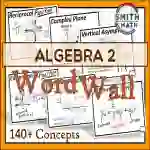Math > Algebra | Grade 10, 11, 12 | Graphics

# Algebra 2 Word WallAttributes
Subject

Algebra

Types

Graphics

File

PDF

Editable
No
Author
RatingThis is an Algebra 2 Word Wall with over 140 concepts included.

You are able to easily click on the topics and be skipped to that section within the PDF.

You can print off these vocabulary cards and laminate them to displayed on your word wall.

The topics included are:

1. Linear Equations & Inequalities

– Invisible Numbers

– Linear Equations: SlopeIntercept, Point Slope, & Standard Form

– Slope Formula

– Graphing 2-Variable Inequalities

2. Systems of Equations

– System of Equations

– Solving Systems: Graphing, Substitution, Elimination

– Solutions of Systems with 2 Variables: 1 Solution, No Solution, Infinite Solution

– Solutions of Systems with 3 Variables: 1 Solution, No Solution, Infinite Solution

– System of Inequalities

– Quadratic Functions: Vertex Form, Standard Form, & Factored/Intercept Form

– Parabolas: Max, Min, Axis of Symmetry

– Complete the Square

– Zero Product Property

– Solving Quadratic Equations Graphic Organizer

– Discriminant Graphic Organizer

4. Factoring

– Difference of Squares

– Perfect Square Trinomials

– Sum of Cubes

– Difference of Cubes

5. Complex Numbers

– Complex Numbers: Imaginary Unit, Rewriting with i

– Complex Conjugates

– Operations with Complex Numbers: Adding/Subtracting, Multiplying, & Dividing

– Complex Plane

6. Composition of Functions & Inverses

– Vertical Line Test

– Function Operations

– Composition of Functions

– Inverses: Relation, Equation, & Graph

7. Polynomial Functions

– Polynomials: Degree, Leading Coefficient, Naming

– Zeros of Polynomials: Factors, Zeros/Roots, & x-intercepts

– End Behavior

– Long Division

– Synthetic Division

– Fundamental Thm of Algebra

– Factor Theorem

– Remainder Theorem

– Repeated Zeros

– Rational Root Thm

– Conjugate Root Thm

– Descartes’ Rule of Signs

– Binomial Theorem

– Rationalize the Denominator

– Rational Exponents

– Finding nth Roots

– Simplifying nth Roots

9. Exponentials & Logarithms

– Exponential & Logarithmic Form

– Exponential Growth

– Exponential Decay

– Compounded Interest: Annual, Periodic, & Continuous

– Properties of Logs: Product Rule, Quotient Rule, Power Rule, Change of Base Formula

10. Rational Functions

– Direct Variation

– Inverse Variation

– Rational Functions

– Vertical Asymptotes

– Horizontal Asymptotes

– Solving Rational Equations

11. Matrices

– Matrix

– Matrix Subtraction

– Scalar Multiplication

– Matrix Multiplication

– Identity Matrix

– Systems with Matrices

– Determinants: 2x2 and 3x3 Matrices

– Inverse of 2x2 Matrix

– Singular Matrix

12. Conic Sections

– Parabolas

– Ellipses

– Hyperbolas

– Circles

13. Sequences & Series

– Arithmetic Sequence: Pattern, Recursive Formula, Explicit Formula

– Geometric Sequence: Pattern, Recursive Formula, Explicit Formula

– Geometric Series: Finite & Infinite

– Arithmetic Series

14. Key Features of Functions

– Tables & Graphs: Linear, Quadratic, & Exponential Functions

– Absolute Value Functions

– Square Root Functions

– Exponential Function

– Logarithmic Function

– Reciprocal Function

## What's Included

A 213 page printable PDF

## Resource Tags

math graphics bulletin boards math clip art algebra 2 vocabulary terms algebra 2 word wall math word wall algebra posters math vocabualry algebra anchor charts

## 0 Reviews

### you may also like...

Check out these other great products